# SSAT Upper Level Math : How to find if acute / obtuse triangles are congruent

## Example Questions

### Example Question #1 : Acute / Obtuse Triangles

If the vertex angle of an isosceles triangle is 30 degrees, what is the value of the angle that is exterior to one of the base angles?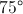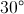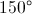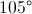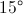First, you subtract one angle so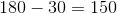. Because there are 2 base angles you divide that value by 2 to get 75 Degrees. The exterior angle and the base angle are supplementary angles so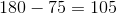.